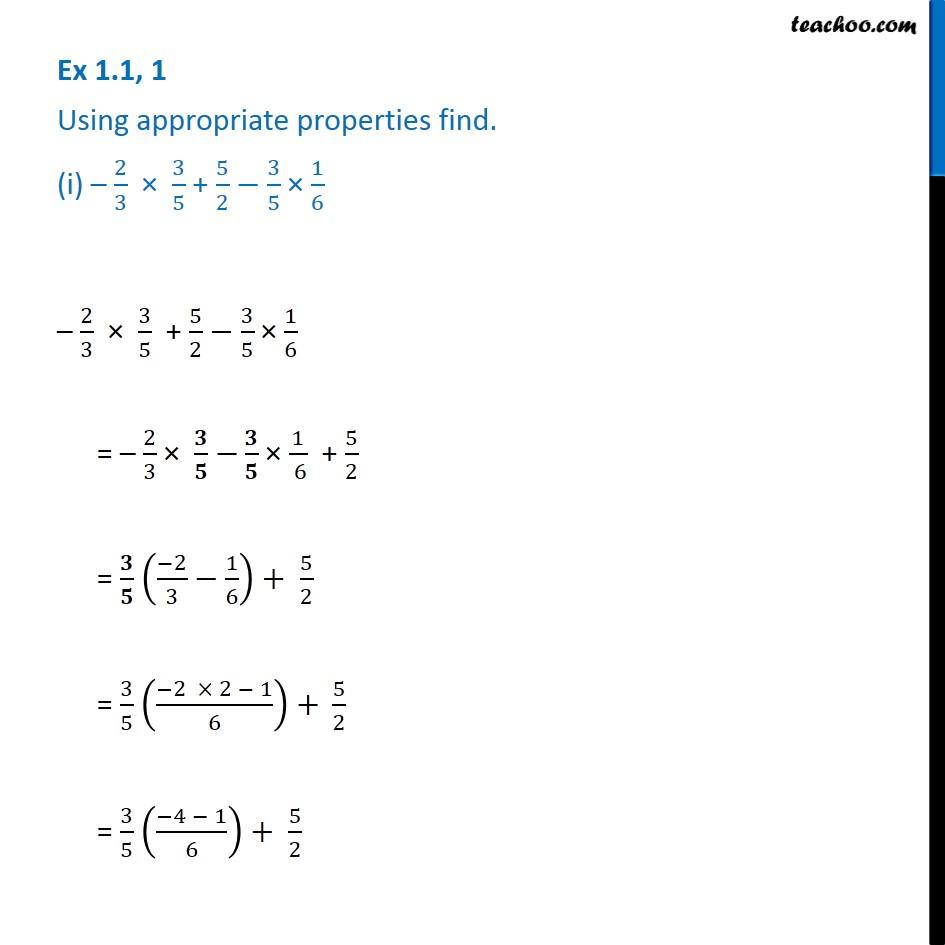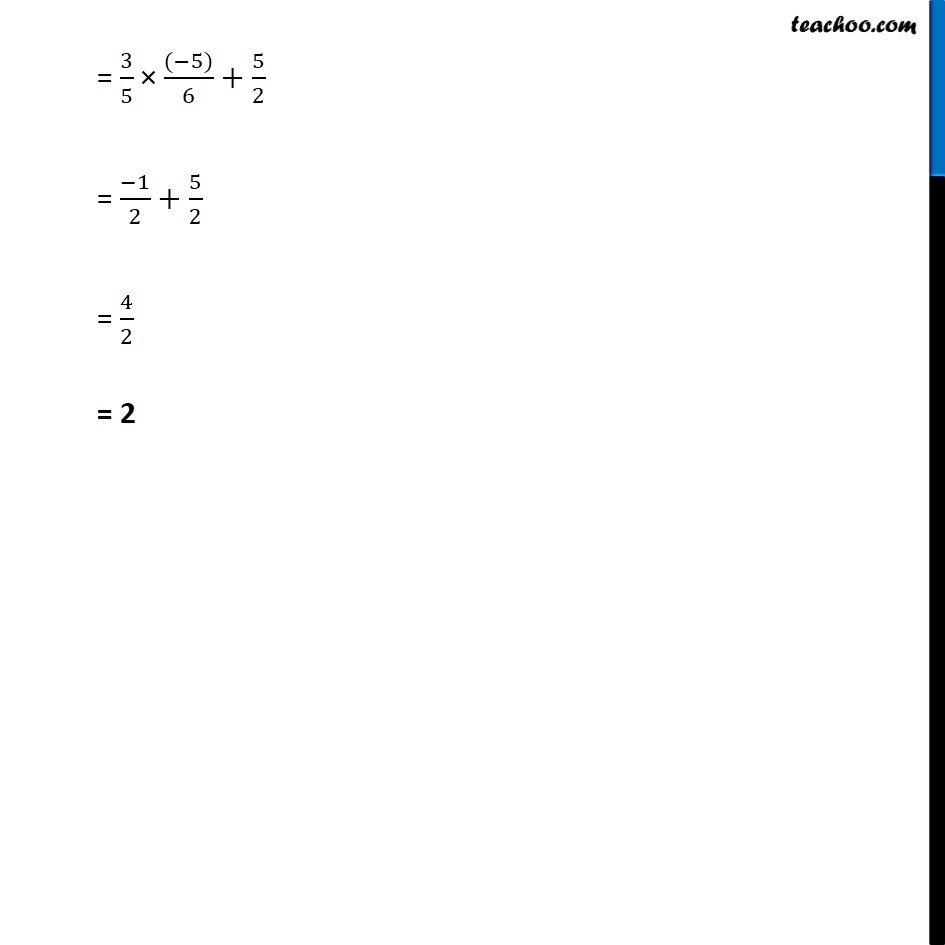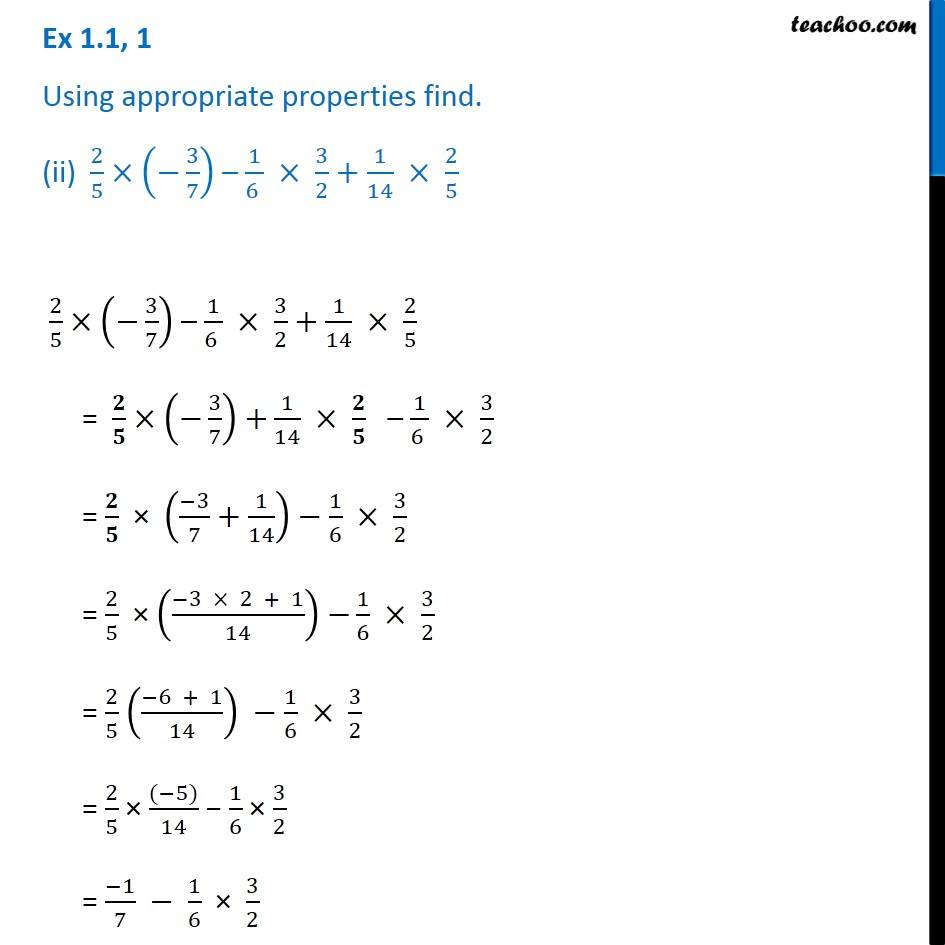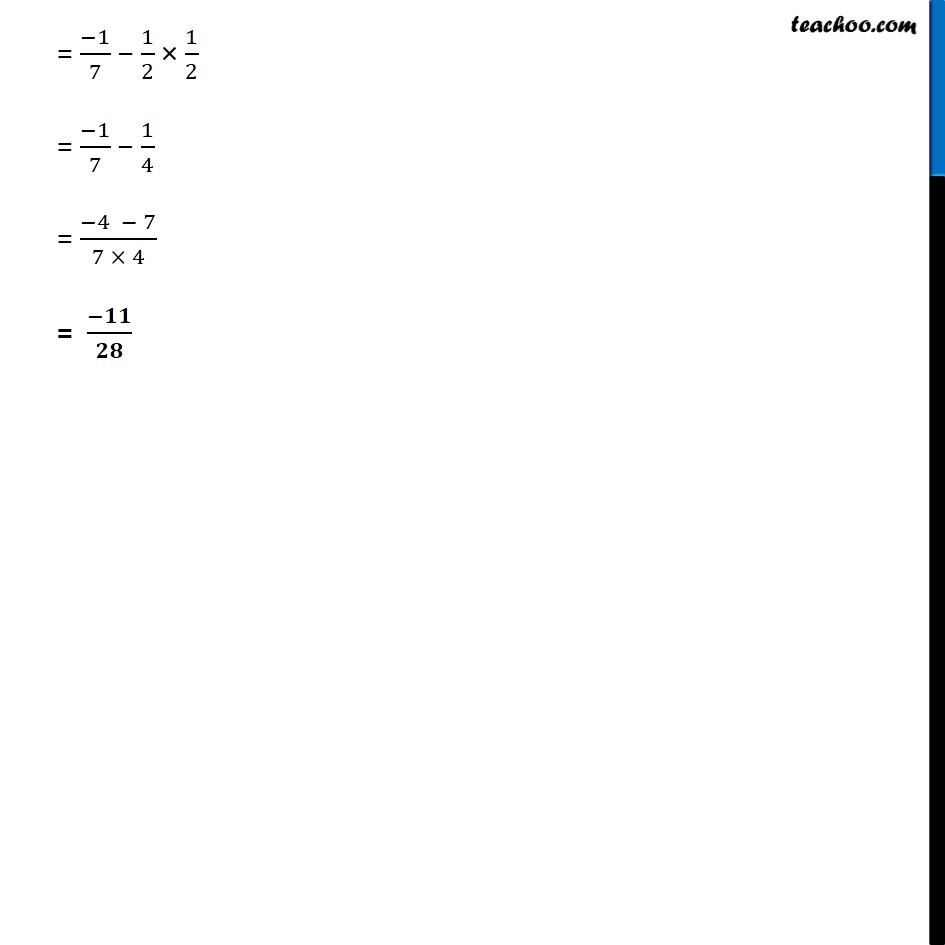1. Chapter 1 Class 8 Rational Numbers
2. Serial order wise
3. Ex 1.1

Transcript

Ex 1.1, 1 Using appropriate properties find. (i) – 2/3 × 3/5 + 5/2−3/5 × 1/6 – 2/3 × 3/5 + 5/2−3/5 × 1/6 = – 2/3 × 𝟑/𝟓 −𝟑/𝟓 × 1/( 6) + 5/2 = 𝟑/𝟓 ((−2)/3−1/6)+ 5/2 = 3/5 ((−2 × 2 − 1)/6) + 5/2 = 3/5 ((−4 − 1)/6)+ 5/2 = 3/5 × ((−5))/6+ 5/2 = (−1)/2+5/2 = 4/2 = 2 Ex 1.1, 1 Using appropriate properties find. (ii) 2/5×(−3/7) – 1/(6 ) × 3/2+1/14 × 2/5 2/5×(−3/7) – 1/(6 ) × 3/2+1/14 × 2/5 = 𝟐/𝟓× (−3/7) +1/14 × 𝟐/𝟓 – 1/(6 ) × 3/2 = 𝟐/𝟓 × ((−3)/7+1/14)−1/6 × 3/2 = 2/5 × ((−3 × 2 + 1)/14) −1/6 × 3/2 = 2/5 ((−6 + 1)/14) −1/6 × 3/2 = 2/5 × ((−5))/14 − 1/6 × 3/2 = (−1)/7 − 1/6 × 3/2 = (−1)/7 − 1/2 × 1/2 = (−1)/7 − 1/4 = (−4 − 7)/(7 × 4) = (−𝟏𝟏)/𝟐𝟖

Ex 1.1